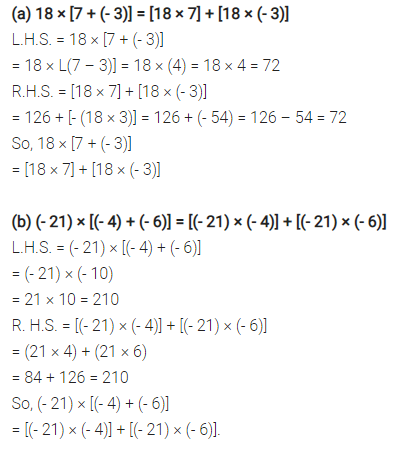# Verify the following: (a) 18 × [7 + (- 3)] = [18 × 7] + [18 × (- 3)]

Verify the following:
(a) 18 × [7 + (- 3)] = [18 × 7] + [18 × (- 3)]
(b) (-21)×[(-4) + (-6)] = [(-21) × (-4)] + [(-21) × (-6)